NCERT Solutions for Class 10 Maths Chapter 13 Surface Areas and Volumes Ex 13.1 are part of NCERT Solutions for Class 10 Maths. Here we have given NCERT Solutions for Class 10 Maths Chapter 13 Surface Areas and Volumes Ex 13.1.

 Board CBSE Textbook NCERT Class Class 10 Subject Maths Chapter Chapter 13 Chapter Name Surface Areas and Volumes Exercise Ex 13.1 Number of Questions Solved 9 Category NCERT Solutions

## NCERT Solutions for Class 10 Maths Chapter 13 Surface Areas and Volumes Ex 13.1

Unless stated otherwise, take π = $$\frac { 22 }{ 7 }$$

13.1 Class 10 Question 1.
2 cubes each of volume 64 cm3 are joined end to end. Find the surface area of the resulting cuboid.Solution:
Volume of one cube = 64 cm3
Let edge of one cube = a
Volume of the cube = (edge)3
a3 = 64 ⇒ a = 4 cm
Similarly, edge of the another cube = 4 cm.
Now, both cubes are joined together and a cuboid is formed as shown in the figure.
Now, length of the cuboid (l) = 8 cm
breadth of the cuboid (b) = 4 cm
height of the cuboid (h) = 4 cm
Surface area of the cuboid so formed = 2 (lb + bh + hl)
= 2(8 x 4 + 4 x 4 + 4 x 8)
= 2(32 + 16 + 32) = 160 cm²

NCERT Class 10 Exercise 13.1 Question 2.
A vessel is in the form of a hollow hemisphere mounted by a hollow cylinder. The diameter of the hemisphere is 14 cm and the total height of the vessel is 13 cm. Find the inner surface area of the vessel.Solution:
Given: diameter of the hemisphere = 14 cm
Radius = $$\frac { 14 }{ 2 }$$ = 7 cm
Curved surface area of the hemisphere = 2πr² = 2 x $$\frac { 22 }{ 7 }$$ x 7 x 7 cm²
= 14 x 22 cm² = 308 cm²
Here, total height of the vessel = 13 cm
Height of the cylinder = Total height – Height of the hemisphere = 13 cm – 7 cm = 6 cm
and radius of the cylinder = radius of the hemisphere = 7 cm
Inner surface area of the cylinder = 2πrh = 2 x $$\frac { 22 }{ 7 }$$ x 7 x 6
= 2 x 22 x 6 = 264 cm²
Inner surface area of the vessel = Inner surface area of the cylinder + curved surface area of the hemisphere
= 264 cm² + 308 cm² = 572 cm²

Ex 13.1 Class 10 Question 3.
A toy is in the form of a cone of radius 3.5 cm mounted on a hemisphere of same radius. The total height of the toy is 15.5 cm. Find the total surface area of the toy.
Solution:Surface Area And Volume Class 10 Question 4.
A cubical block of side 7 cm is surmounted by a hemisphere. What is the greatest diameter the hemisphere can have? Find the surface area of the solid.
Solution: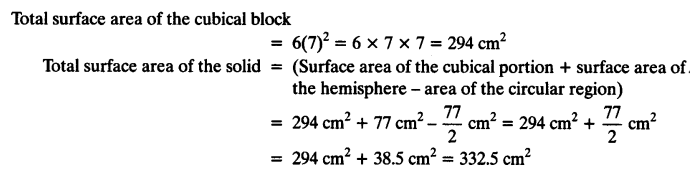Class 10 Maths Chapter 13 Question 5.
A hemispherical depression is cut out from one face of a cubical wooden block such that the diameter l of the hemisphere is equal to the edge of the cube. Determine the surface area of the remaining solid.
Solution:Chapter 13 Class 10 Maths Question 6.
A medicine capsule is in the shape of a cylinder with two hemispheres stuck to each of its ends (see figure). The length of the entire capsule is 14 mm and the diameter of the capsule is 5 mm. Find its surface area.Solution: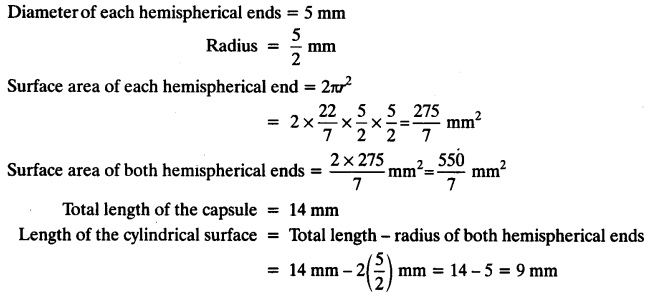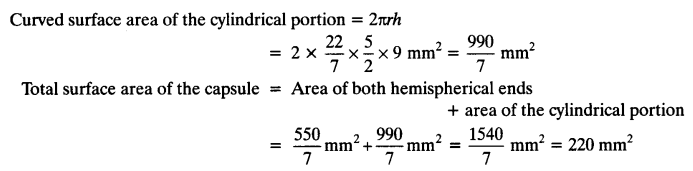Class 10 Maths Chapter 13 Question 7.
A tent is in the shape of a cylinder surmounted by a conical top. If the height and diameter of the cylindrical part are 2.1 m and 4 m respectively, and the slant height of the top is 2.8 m, find the area of the canvas used for making the tent. Also, find the cost of the canvas of the tent at the rate of ₹ 500 per m². (Note that the base of the tent will not be covered with canvas.)
Solution: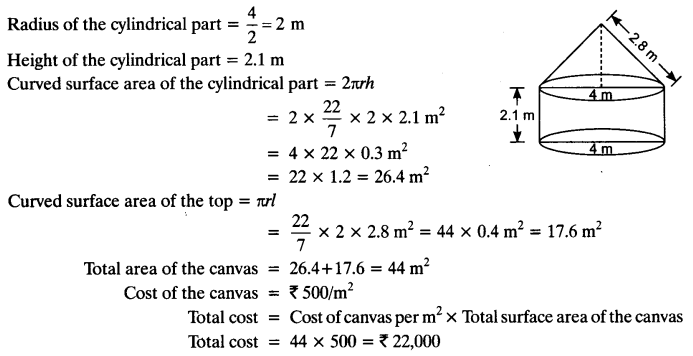Exercise 13.1 Class 10 NCERT Solutions Question 8.
From a solid cylinder whose height is 2.4 cm and diameter 1.4 cm, a conical cavity of the same height and same diameter is hollowed out. Find the total surface area of the remaining solid to the nearest cm².
Solution: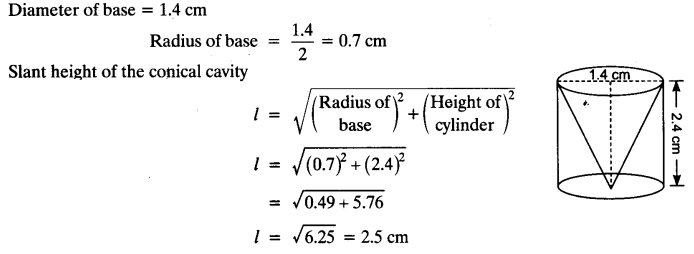Class 10 Maths Chapter 13 Solutions Question 9.
A wooden article was made by scooping out a hemisphere from each end of a solid cylinder, as shown in figure. If the height of the cylinder is 10 cm, and its base is of radius 3.5 cm, find the total surface area of the article.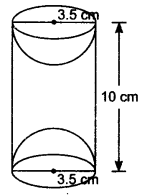Solution:We hope the NCERT Solutions for Class 10 Maths Chapter 13 Surface Areas and Volumes Ex 13.1, help you. If you have any query regarding NCERT Solutions for Class 10 Maths Chapter 13 Surface Areas and Volumes Ex 13.1, drop a comment below and we will get back to you at the earliest.